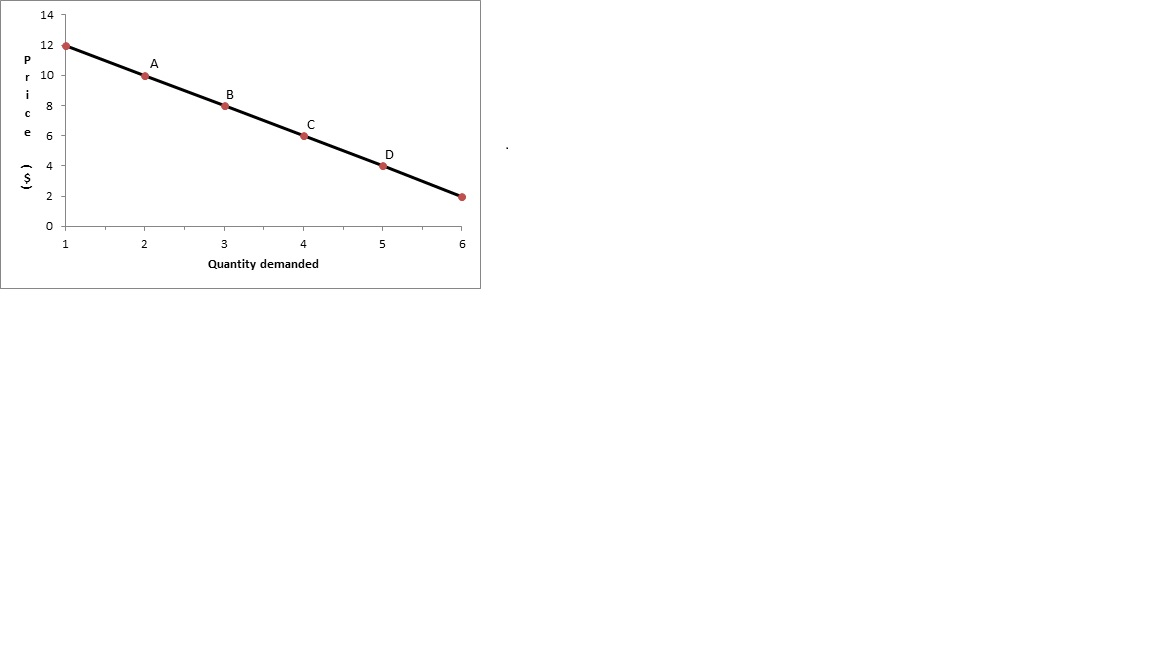# AP Microeconomics : Short-run Earnings

## Example Questions

### Example Question #1 : Short Run Earnings

Use the following graph for questions 9 - 11Increasing the price of oranges at point D will result in:

1. An increase in total revenue
2. A decrease in quantity demanded
3. Movement toward a portion of the demand curve that is more elastic

3 only

2 only

1, 2, and 3

1 and 2

1 only

1, 2, and 3

Explanation:

If we are in the inelastic portion of the demand curve, an increase in price will increase TR, since the price effect is greater than the quantity effect. Quantity will still decrease.

### Example Question #12 : Microeconomics Graphs

Use the following graph to answer questions 9-11:What is the total revenue generated at point A?

30

10

12

20

24